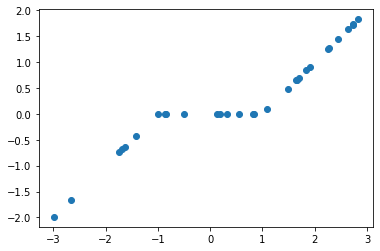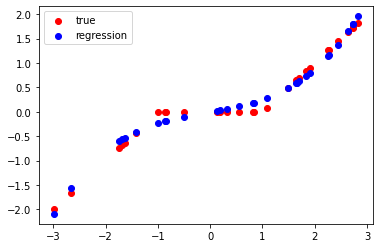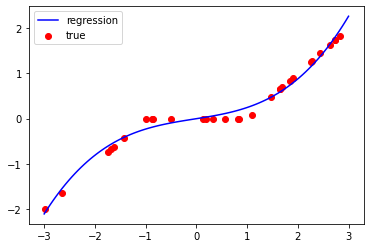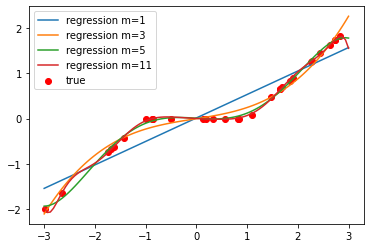# 4- Exercises¶

Exercise 1: In polynomial regression, we are given $n$ points $(x_i,y_i)$ and fit a size-$m$ polynome $f(x) = c_0 + c_1 x + c_2 x^2 + .. + c_m x^m$ so that $f(x_i)\approx y_i$ for all $i=1,..n$.

In this exercise, we want to find the $m+1$ coefficients $(c_j)$ that minimize the least square error $$\left\| ~~~ \underbrace{ \left[ \begin{array}{ccccc} 1 & x_1 & x_1^2 & .. & x_1^m \\ 1 & x_2 & x_2^2 & .. & x_2^m \\ \vdots & \vdots & \vdots & \vdots & \vdots \\ 1 & x_n & x_n^2 & .. & x_n^m \end{array} \right] }_{X} ~ \underbrace{ \left[ \begin{array}{c} c_0 \\ c_1 \\ c_2 \\ \vdots \\ c_m \end{array} \right] }_{c} - \underbrace{ \left[ \begin{array}{c} y_1 \\ y_2 \\ \vdots \\ y_n \end{array} \right] }_{y} \right\|^2$$

1. Construct the matrix $X$ from the given vector $x$ below with $m=3$.
• Find the optimal $c$ by solving the least square problem $\min_c \|Xc-y\|^2$ using Numpy (lstsq and solve). Is the result exact (no error)?
• Plot the obtained polynome on the scatter plot.
• Redo questions 2-3 by varying the relative sizes of $n$ and $m$. What do you observe?
In :
import numpy as np

n = 30 # Number of sample points

x = (np.random.rand(n)-0.5)*6
y = np.sign(x)*np.maximum(np.abs(x)-1.0,0.0)

In :
import matplotlib.pyplot as plt

plt.scatter(x,y)

Out:
<matplotlib.collections.PathCollection at 0x7f0bbd7c8580>In :
m = 3

X = np.array( [[e**i for i in range(m+1)] for e in x] ) # Construction of the matrix X
#               e**i for i=0,1,2,..,m and e going through the coordinates of x

In :
X

Out:
array([[ 1.00000000e+00,  2.63475918e+00,  6.94195594e+00,
1.82903821e+01],
[ 1.00000000e+00,  1.08742164e+00,  1.18248583e+00,
1.28586068e+00],
[ 1.00000000e+00, -8.52882539e-01,  7.27408625e-01,
-6.20394114e-01],
[ 1.00000000e+00, -8.67288848e-01,  7.52189946e-01,
-6.52365952e-01],
[ 1.00000000e+00, -9.91439323e-01,  9.82951932e-01,
-9.74537198e-01],
[ 1.00000000e+00, -2.65539394e+00,  7.05111697e+00,
-1.87234933e+01],
[ 1.00000000e+00, -1.73681628e+00,  3.01653078e+00,
-5.23915976e+00],
[ 1.00000000e+00,  1.69509454e+00,  2.87334548e+00,
4.87059223e+00],
[ 1.00000000e+00,  8.26510199e-01,  6.83119109e-01,
5.64604911e-01],
[ 1.00000000e+00, -1.68268993e+00,  2.83144540e+00,
-4.76444467e+00],
[ 1.00000000e+00,  1.90777691e+00,  3.63961276e+00,
6.94356920e+00],
[ 1.00000000e+00,  2.72749084e+00,  7.43920627e+00,
2.02903670e+01],
[ 1.00000000e+00,  8.43714652e-01,  7.11854415e-01,
6.00602000e-01],
[ 1.00000000e+00,  1.64969298e+00,  2.72148692e+00,
4.48961786e+00],
[ 1.00000000e+00,  5.59650926e-01,  3.13209159e-01,
1.75287796e-01],
[ 1.00000000e+00,  2.44432829e+00,  5.97474078e+00,
1.46042279e+01],
[ 1.00000000e+00, -2.99125698e+00,  8.94761834e+00,
-2.67646259e+01],
[ 1.00000000e+00,  1.65388275e+00,  2.73532815e+00,
4.52391204e+00],
[ 1.00000000e+00,  2.83004819e+00,  8.00917277e+00,
2.26663449e+01],
[ 1.00000000e+00, -1.63054273e+00,  2.65866958e+00,
-4.33507434e+00],
[ 1.00000000e+00,  2.25922805e+00,  5.10411137e+00,
1.15313516e+01],
[ 1.00000000e+00,  1.84178373e+00,  3.39216730e+00,
6.24763852e+00],
[ 1.00000000e+00,  1.35923759e-01,  1.84752682e-02,
2.51122789e-03],
[ 1.00000000e+00, -5.07120413e-01,  2.57171113e-01,
-1.30416721e-01],
[ 1.00000000e+00,  2.27485856e+00,  5.17498146e+00,
1.17723509e+01],
[ 1.00000000e+00,  2.73132410e+00,  7.46013133e+00,
2.03760365e+01],
[ 1.00000000e+00, -1.42589364e+00,  2.03317269e+00,
-2.89908801e+00],
[ 1.00000000e+00,  1.80942767e-01,  3.27402850e-02,
5.92411777e-03],
[ 1.00000000e+00,  1.48135295e+00,  2.19440655e+00,
3.25069062e+00],
[ 1.00000000e+00,  3.29125824e-01,  1.08323808e-01,
3.56521625e-02]])
In :
res = np.linalg.lstsq(X,y) # we solve the least square problem \min_c \|Xc-y\|^2

/tmp/ipykernel_25543/3084860011.py:1: FutureWarning: rcond parameter will change to the default of machine precision times max(M, N) where M and N are the input matrix dimensions.
To use the future default and silence this warning we advise to pass rcond=None, to keep using the old, explicitly pass rcond=-1.
res = np.linalg.lstsq(X,y) # we solve the least square problem \min_c \|Xc-y\|^2

In :
res # res is a tuple containing the solution and other stuff (see the doc)

Out:
(array([-0.0026348 ,  0.1771313 ,  0.00886646,  0.06127616]),
array([0.40635888]),
4,
array([60.28839564, 21.49126616,  3.9382577 ,  3.43012419]))
In :
c = res # the solution c is the first element of res

In :
y_regression = X.dot(c) # Xc is the obtained regression value for the points

In :
plt.scatter(x,y,color='r',label='true')
plt.scatter(x,y_regression,color='b',label='regression')
plt.legend()

Out:
<matplotlib.legend.Legend at 0x7f0bb565dac0>In :
# If I want to see better what happens, I can generate 100 points in [-3,3] and compute the regression output with the optimal c
x_lin = np.linspace(-3,3,100)
X_lin = np.array( [[e**i for i in range(m+1)] for e in x_lin] )
y_pred = X_lin.dot(c)

In :
plt.scatter(x,y,color='r',label='true')
plt.plot(x_lin,y_pred,color='b',label='regression') # I use a plot to show a solid line
plt.legend()

Out:
<matplotlib.legend.Legend at 0x7f0bb5646d30>In :
x_lin = np.linspace(-3,3,100)

for m in [1,3,5,11]: # I do the same above for multiple values of m
X = np.array( [[e**i for i in range(m+1)] for e in x] )
c = np.linalg.lstsq(X,y,rcond=None);

X_lin = np.array( [[e**i for i in range(m+1)] for e in x_lin] )
y_pred = X_lin.dot(c)

plt.plot(x_lin,y_pred,label='regression m={}'.format(m))

plt.scatter(x,y,color='r',label='true')
plt.legend()

Out:
<matplotlib.legend.Legend at 0x7f0bb5613670>